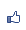TiKZ 的常用路徑（path）
2015/04/15 14:40:39瀏覽899｜回應0｜推薦0

1. 格子。使用grid命令，用法是 （起點座標）grid （終點坐標）。例如：

\draw (0,0) grid (5,5); 就是畫出一個從(0,0) 到 (5,5)的格子，TiKz預設的單位是公分。grid 命令有幾個常用參數：

step, xstep, ystep, help lines。

step=0.5cm，就是每0.5公分為一單位的格子，

xstep, ystep, 對應到x, y方向的間格單位。

help lines，是畫出極細，灰色的格線，方便作圖。

\draw (0,0) grid (5,5);

\draw[xshift=6cm] (0.1,0.1) grid (4.9,4.9);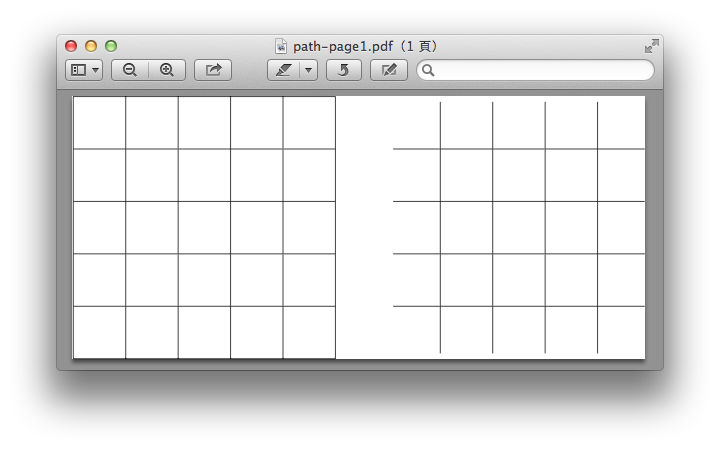\draw[step=0.5cm] (0,0) grid (5,5);

\draw[xshift=6cm,step=0.5cm] (0.1,0.1) grid (4.9,4.9);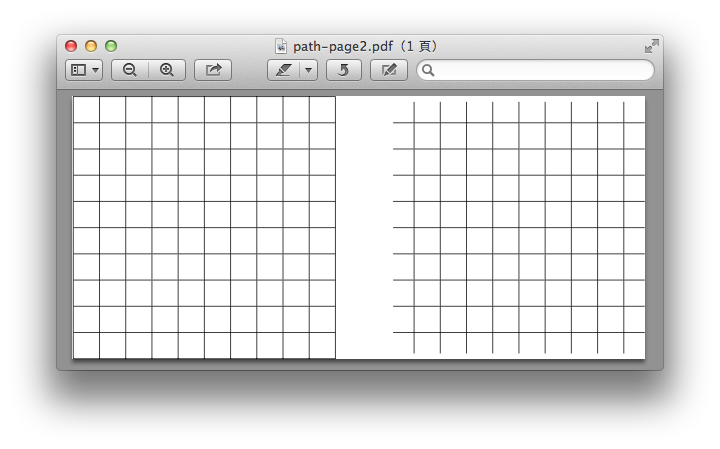\draw [xstep=0.5cm](0,0) grid (5,5);

\draw [xstep=0.5cm,xshift=6cm, help lines] (0,0) gird (5,5);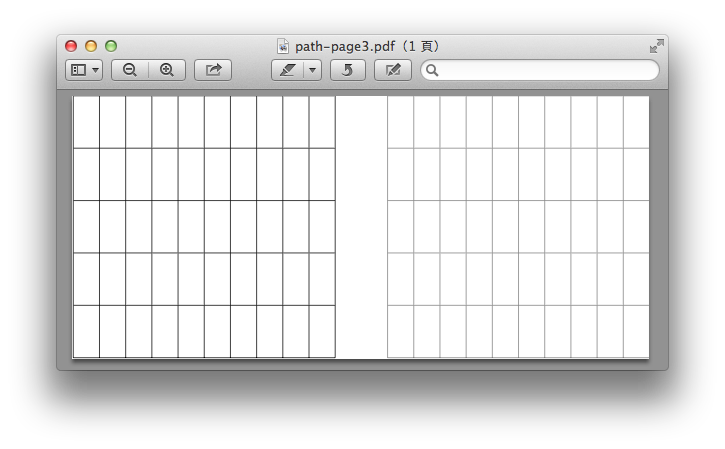2. 距形，用rectangle指令：（起點）rectangle （終點）

\draw (0,0) rectangle (2,1);

\draw [red](-1,-1) rectangle (1,3);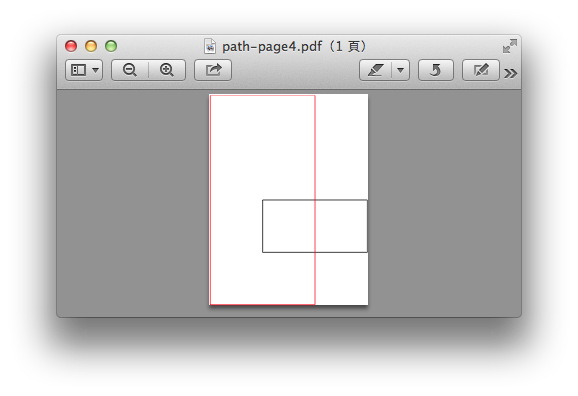3.圓，橢圓。畫圖路徑用circle指令，畫橢圓路徑用ellipse指令。圓路徑的用法：

（圓心座標）circle（圓半徑長度）

\draw (0,0) circle (1cm);

\draw [red](0,0) ellipse (2cm and 1cm);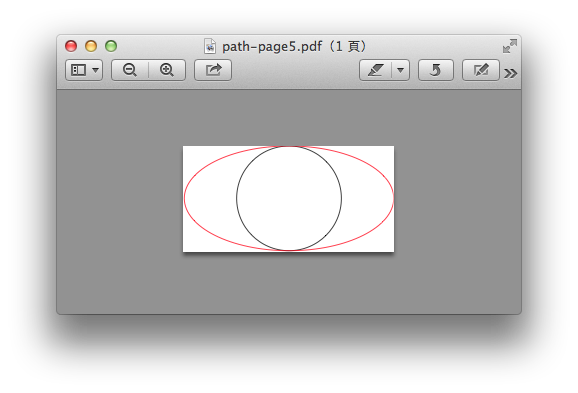4. 弧，使用arc指令，分成圓弧橢圓弧： (弧起點) arc [start angel= 角度值，end angle=角度值，弧半徑（x軸，y軸）長度]

\draw (0,0) arc (start angle =0, end angle=60, radius=1cm);

\draw [blue](0,0) arc (start angle =0, end angel =180, x radius=0.5cm, y radius=1.5cm];

\draw (0,0) arc [start angle =180, delta angle=90, radius=1cm];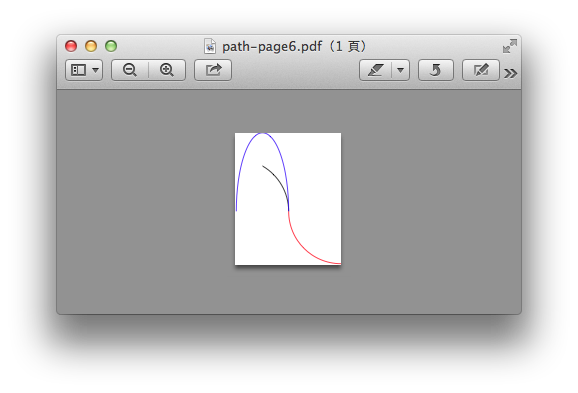5.抛物線parabola，用法（起始點） parabola  bend (最高點座標)  （終點座標）

\draw [help lines] (0,0) grid (2,3);

\draw (0,0) parabola  (2,1);

draw [red](0,0) parabola bend (1,3) (2,2);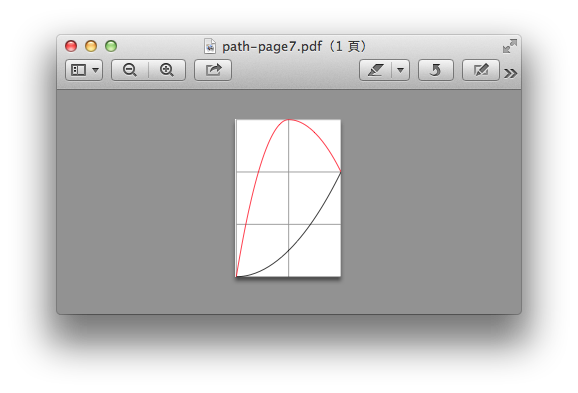6. 正弦sine餘弦cosine。用法：(起點座標) sin（終點座標）；(起點座標) cos（終點座標）。前後二點是畫出0度到90度的sine或cosine曲線。

\draw (0,1) cos (1,0) cos (2,-1) sin (4,0.5);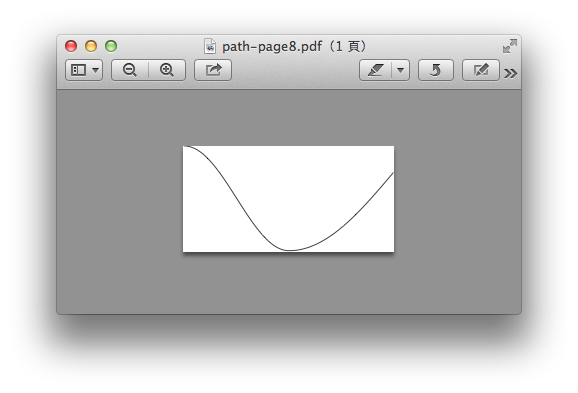( 知識學習科學百科 )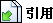引用網址：https://classic-blog.udn.com/article/trackback.jsp?uid=paraquat&aid=22252899